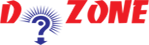﻿ How to find the Square Root?## Python Program to Calculate the Area of a Triangle

Here in this program, we will tell you about how to calculate the area of triangle and display it. If x, y and z are the three sides of a triangle. Then,

``````
s = (x+y+z) / 2
area = √(s(s-a)*(s-b)*(s-c))
```
```
``````
Input
# Python Program to find the area of triangle

a = 5
b = 6
c = 7

# Uncomment below to take inputs from the user
# a = float(input('Enter first side: '))
# b = float(input('Enter second side: '))
# c = float(input('Enter third side: '))

# calculate the semi-perimeter
s = (a + b + c) / 2

# calculate the area
area = (s*(s-a)*(s-b)*(s-c)) ** 0.5
print('The area of the triangle is %0.2f' %area)
Output

The area of the triangle is 14.70
```
```

Conclusion: Here by using Heron's formaula, we calculate the area of triangle. But, to calculate the area of a triangle you can use input() function as well.

## Other Python Programs

PythonTraining, 258-FF , Katewa Nagar, New Sanganer Rd, Jaipur, 302019